## 2012年5月7日 星期一

### [Python Std Library] Numeric and Math Modules : operator — Standard operators as functions

Preface :
The operator module exports a set of efficient functions corresponding to the intrinsic operators of Python. For example, operator.add(x, y) is equivalent to the expression x+y. The function names are those used for special class methods; variants without leading and trailing __ are also provided for convenience.

Comparison operations :
The functions fall into categories that perform object comparisons, logical operations, mathematical operations, sequence operations, and abstract type tests. The object comparison functions are useful for all objects, and are named after the rich comparison operators they support :
operator.lt(a, b)/operator.__lt__(a, b)
operator.le(a, b)/operator.__le__(a, b)
operator.eq(a, b)/operator.__eq__(a, b)
operator.ne(a, b)/operator.__ne__(a, b)
operator.ge(a, b)/operator.__ge__(a, b)
operator.gt(a, b)/operator.__gt__(a, b)
New in version 2.2.
Perform "rich comparisons" between a and b. Specifically, lt(a, b) is equivalent to a < ble(a, b) is equivalent to a <= beq(a, b) is equivalent to a == bne(a, b) is equivalent to a != bgt(a, b) is equivalent to a > b and ge(a, b) is equivalent to a >= b. Note that unlike the built-in cmp(), these functions can return any value, which may or may not be interpretable as a Boolean value. See Comparisons for more information about rich comparisons.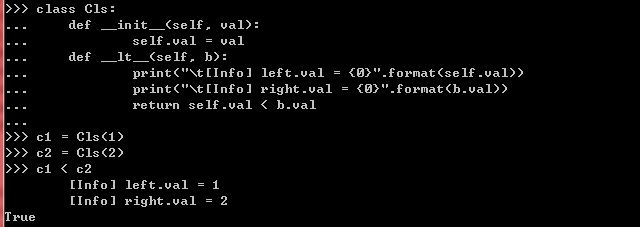Logical operations :
The logical operations are also generally applicable to all objects, and support truth tests, identity tests, and boolean operations :
operator.not_(obj)/operator.__not__(obj)
Return the outcome of not obj. (Note that there is no __not__() method for object instances; only the interpreter core defines this operation. The result is affected by the __nonzero__() and __len__() methods.)

The mathematical and bitwise operations :
operator.abs(obj)/operator.__abs__(obj)
Return the absolute value of obj :Return a + b, for a and b numbers :operator.and_(a, b)/operator.__and__(a, b)
Return the bitwise and of a and b :operator.div(a, b)/operator.__div__(a, b)
Return a / b when __future__.division is not in effect. This is also known as "classic" division :operator.floordiv(a, b)/operator.__floordiv__(a, b)
New in version 2.2.
Return a // b.

operator.index(a)/operator.__index__(a)
New in version 2.5.
Return a converted to an integer. Equivalent to a.__index__().

operator.invert(obj)/operator.__invert__(obj)
Return the bitwise inverse of the number obj. This is equivalent to ~obj :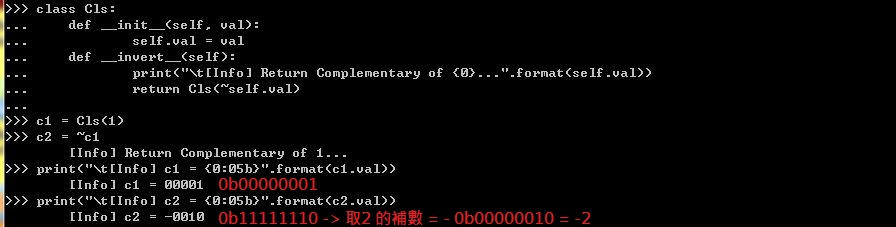operator.lshift(a, b)/operator.__lshift__(a, b)
Return a shifted left by b :operator.mod(a, b)/operator.__mod__(a, b)
Return a % b.

operator.mul(a, b)/operator.__mul__(a, b)
Return a * b, for a and b numbers.

operator.neg(obj)/operator.__neg__(obj)
Return obj negated (-obj).

operator.or_(a, b)/operator.__or__(a, b)
Return the bitwise or of a and b.

operator.pos(obj)/operator.__pos__(obj)
Return obj positive (+obj).

operator.pow(a, b)/operator.__pow__(a, b)
New in version 2.3.
Return a ** b, for a and b numbers.

operator.rshift(a, b)/operator.__rshift__(a, b)
Return a shifted right by b.

operator.sub(a, b)/operator.__sub__(a, b)
Return a - b :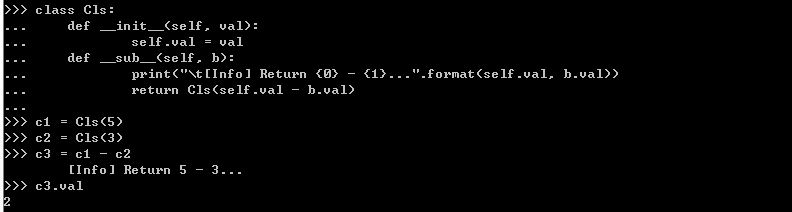operator.truediv(a, b)/operator.__truediv__(a, b)
New in version 2.2.
Return a / b when __future__.division is in effect. This is also known as “true” division :Sequences operations :
Operations which work with sequences (some of them with mappings too) include :
operator.concat(a, b)/operator.__concat__(a, b)
Return a + b for a and b sequences :operator.contains(a, b)/operator.__contains__(a, b)
New in version 2.0: The name __contains__().
Return the outcome of the test b in a. Note the reversed operands :operator.getitem(a, b)/operator.__getitem__(a, b)
Return the value of a at index b :operator.setitem(a, b, c)/operator.__setitem__(a, b, c)
Set the value of a at index b to c :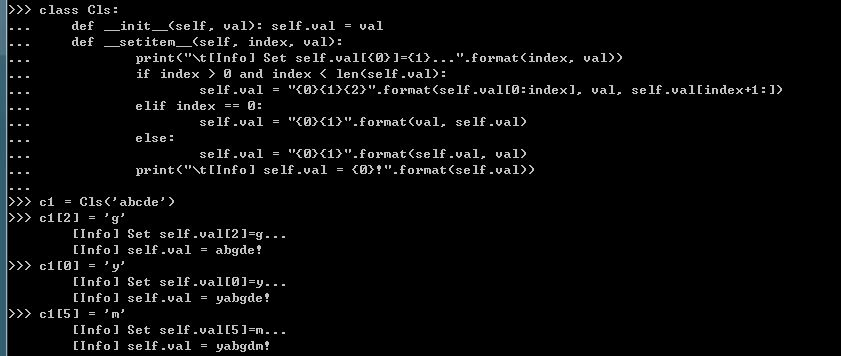In-Place Operations :
Many operations have an "in-place" version. The following functions provide a more primitive access to in-place operators than the usual syntax does; for example, thestatement x += y is equivalent to x = operator.iadd(x, y). Another way to put it like that z = operator.iadd(x, y) is equivalent to the compound statement z = x; z += y
New in version 2.5.
a = iadd(a, b) is equivalent to a += b :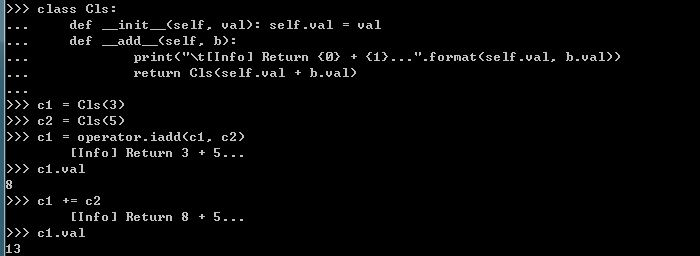Predicate Functions :
The operator module also defines a few predicates to test the type of objects; however, these are not all reliable. It is preferable to test abstract base classes instead (see collections and numbers for details).
operator.isCallable(obj)
Deprecated since version 2.0: Use isinstance(x, collections.Callable) instead.
Returns true if the object obj can be called like a function, otherwise it returns false. True is returned for functions, bound and unbound methods, class objects, and instance objects which support the __call__() method.

operator.isMappingType(obj)
Deprecated since version 2.7: Use isinstance(x, collections.Mapping) instead.
Returns true if the object obj supports the mapping interface. This is true for dictionaries and all instance objects defining __getitem__().

operator.isNumberType(obj)
Deprecated since version 2.7: Use isinstance(x, numbers.Number) instead.
Returns true if the object obj represents a number. This is true for all numeric types implemented in C.

operator.isSequenceType(obj)
Deprecated since version 2.7: Use isinstance(x, collections.Sequence) instead.
Returns true if the object obj supports the sequence protocol. This returns true for all objects which define sequence methods in C, and for all instance objects defining __getitem__().

Fields Extraction :
The operator module also defines tools for generalized attribute and item lookups. These are useful for making fast field extractors as arguments for map()sorted(),itertools.groupby(), or other functions that expect a function argument.
operator.attrgetter(attr[, args...])
New in version 2.4.
Changed in version 2.5: Added support for multiple attributes.
Changed in version 2.6: Added support for dotted attributes.

Return a callable object that fetches attr from its operand. If more than one attribute is requested, returns a tuple of attributes. After, f = attrgetter('name'), the callf(b) returns b.name. After, f = attrgetter('name', 'date'), the call f(b) returns (b.name, b.date). The attribute names can also contain dots; after f = attrgetter('date.month'), the call f(b) returns b.date.month :operator.itemgetter(item[, args...])
New in version 2.4.
Changed in version 2.5: Added support for multiple item extraction.

Return a callable object that fetches item from its operand using the operand’s __getitem__() method. If multiple items are specified, returns a tuple of lookup values. Return a callable object that fetches item from its operand using the operand’s __getitem__() method. If multiple items are specified, returns a tuple of lookup values. Usage example :operator.methodcaller(name[, args...])
New in version 2.6.
Return a callable object that calls the method name on its operand. If additional arguments and/or keyword arguments are given, they will be given to the method as well. After f = methodcaller('name'), the call f(b) returns b.name(). After f = methodcaller('name', 'foo', bar=1), the call f(b) returns b.name('foo', bar=1) :Mapping Operators to Functions :
This table shows how abstract operations correspond to operator symbols in the Python syntax and the functions in the operator module :Supplement :
[Python 學習筆記] 函式、類別與模組 : 類別 (特殊方法名稱)
Python 3.1 快速導覽 - 位元運算

#### 1 則留言:

1.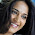I like your blog, I read this blog please update more content on hacking, further check it once at python online course

### [ Py DS ] Ch5 - Machine Learning (Part2)

Source From  Here   Introducing Scikit-Learn   There are several Python libraries that provide solid implementations of a range of machin...